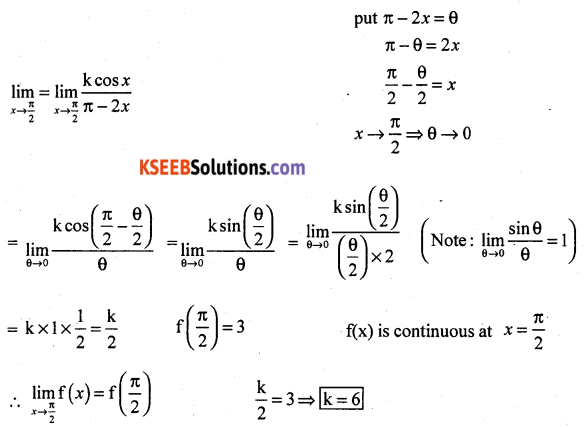# 2nd PUC Maths Previous Year Question Paper March 2017

Students can Download 2nd PUC Maths Previous Year Question Paper March 2017, Karnataka 2nd PUC Maths Model Question Papers with Answers helps you to revise the complete Karnataka State Board Syllabus and score more marks in your examinations.

## Karnataka 2nd PUC Maths Previous Year Question Paper March 2017

Time: 3 Hrs 15 Min
Max. Marks: 100

Instructions

• The question paper has five parts namely A, B, C, D, and E. Answer all the parts.
• Use the graph sheet for the question on Linear programming in Part – E

Part – A

Answer ALL the following questions: (10 × 1 = 10)

Question 1.
Let * be the binary operation on N, given by a * b = LCM of a and b. Find 20 * 16.
Solution:
20 * 16 = LCM of 20 and 16 = 80

Question 2.
Find the principal value of cosec-1 (-√2)
Solution:
cosec-1 (-√2) = -cosec √2 = $$-\pi / 4$$

Question 3.
Construct a 2 × 2 matrix, A = [aij], where elements are given by, aij = $$\frac{i}{j}$$
Solution:
a11 = $$\frac{1}{1}$$ = 1
a12 = $$\frac{1}{2}$$
a21 = $$\frac{2}{1}$$ = 2
a22 = $$\frac{2}{2}$$ = 1
∴ A = $$\left[\begin{array}{ll}1 & 2 \\1 / 2 & 1\end{array}\right]$$Question 4.
If A is a square matrix with |A| = 8 then find the value of |AA’|.
Solution:
|AA’| = |A| |A’| = (8) (8) = 64

Question 5.
If y = cos√x, find $$\frac{d y}{d x}$$
Solution: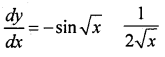Question 6.
Find $$\int\left(\sqrt{x}+\frac{1}{\sqrt{x}}\right) d x$$
Solution: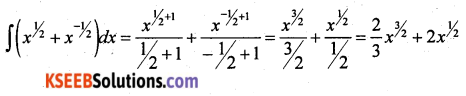Question 7.
Define collinear vectors.
Solution:
Collinear vectors Two or moe non-zero vectors are said to be collinear if they are parallel to the same line. Two vectors a and b are collinear if a = λb for some scalar λ.Question 8.
Find the direction cosines of a line which makes equal angles with the positive co-ordinates axes.
Solution:
α = β = r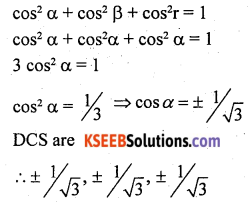Question 9.
Define a feasible region in a linear programming problem.
Solution:
The region containing the set of points satisfying all the constraints of an LPP is called the feasible region.

Question 10.
If A and B are independent events, P(A) = $$\frac{3}{5}$$ and P(B) = $$\frac{1}{5}$$ then find P(A∩B).
Solution:
P(A∩B) = P(A) . P(B) = $$\frac{3}{5} \cdot \frac{1}{5}=\frac{3}{25}$$

Part – B

Answer any TEN questions: (10 × 2 = 20)

Question 11.
If f : R → R defined by f(x) = 1 + x2, then show that f is neither 1-1 nor onto.
Solution:
f(1) = 1 + 12 = 2
f(-1) = 1 + (-1)2 = 2
∴ f(1) = f(-1) But 1 ≠ -1
∴ f is not one-one
Let y ∈ R ∃ x ∈ R such that
f(x) = y
⇒ 1 + x2 = y
⇒ x2 = y – 1
⇒ x = $$\sqrt{y-1}$$
if y = 0 then x = $$\sqrt{-1} \notin \mathrm{R}$$
∴ 0 has no pre-image
∴ f is not onto.Question 12.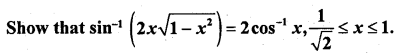Solution: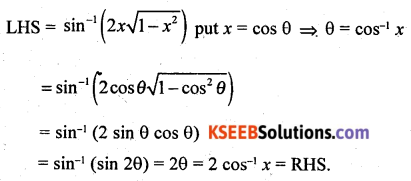Question 13.
Solve the equaton $$\tan ^{-1}\left(\frac{1-x}{1+x}\right)=\frac{1}{2} \tan ^{-1} x,(x>0)$$
Solution: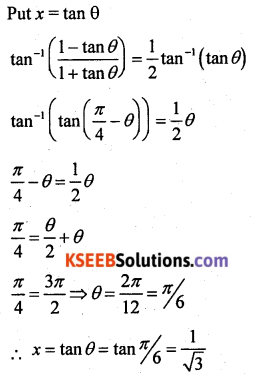Question 14.
Find the values of k, if the area of the triangle is 4 sq. units and vertices are (k, 0), (4, 0) and (0, 2) using determinant.
Solution: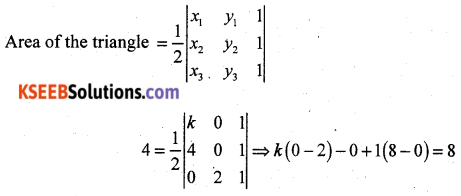⇒ -2k + 8 = 8
⇒ 2k =
⇒ k = 0
on taking -ve sign we get -2k + 8 = -8
⇒ 2k = 16
⇒ k = 8
∴ k = 0, 8.

Question 15.
If ax + by2 = cos y, find $$\frac{d y}{d x}$$
Solution: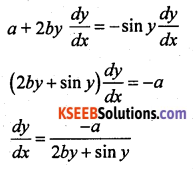Question 16.
Verify Rolle’s theorem for the function f(x) = x2 + 2x – 8, x ∈ [-4, 2]
Solution:
f(x) is a polynomial in x. Flence it is continuous over {-4, 2} and differentiable over (-4, 2).
f(-4) = (-4)2 + 2(-4) – 8 = 16 – 8 – 8 = 0
f(2) = 4 + 4 – 8 = 0
∴ f(-4) = f(2)
∴ All the conditions of the Rolle’s theorem are satisfied.
∴ there exists a c ∈ [-4, 2] such that
f'(c) = 0
f'(x) = 2x + 2
f'(c) = 2c + 2
f'(c) = 0
⇒ 2c + 2 = 0
⇒2c = -2
⇒ c = -1 ∈ [-4, 2]
∴ Rolle’s theorem is verified.Question 17.
Find the approximate change in the volume of a cube of side x metres caused by increasing the side by 3%.
Solution:
v = x3
∆x = 3% of x = (0.03)x
$$\frac{d v}{d x}$$ = 3x2
∆v = $$\frac{d v}{d x}$$ . ∆x
= (3x2) (0.03) x
= 0.09 x3 m3

Question 18.
Integrate $$\frac{\tan ^{4} \sqrt{x} \sec ^{2} \sqrt{x}}{\sqrt{x}}$$ with respect to x.
Solution: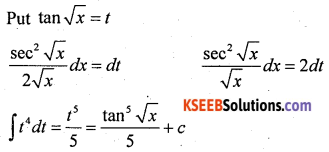Question 19.
Evaluate $$\int_{0}^{2 / 3} \frac{d x}{4+9 x^{2}}$$
Solution: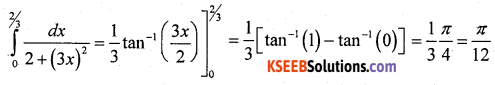Question 20.
Find the order and degree of the differential equation $$\left( \frac { dy }{ dx } \right) ^{ 2 }+\frac { dy }{ dx } { sin }^{ 2 }y=0$$
Solution:
Order = 1, Degree = 2.Question 21.
Find the position vector of a point R which divides the lipe joining two points P and Q whose position vectors are $$\hat{i}+2 \hat{j}-\hat{k}$$ and $$-\hat{i}+\hat{j}+\hat{k}$$ respectively in theratio 2 : 1
(i) Internally.
(ii) Externally.
Solution:
The position vector of a point R divided the line segment joining two points P and Q in the ratio m : n is given by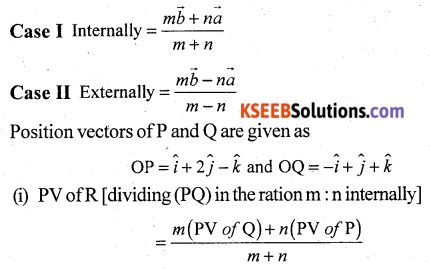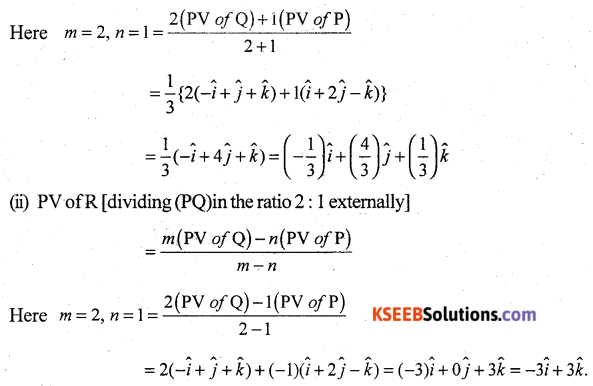Question 22.
Find the area of the parallelogram whose adjacent sides are determined by the vector $$\vec{a}=\hat{i}-\hat{j}+3 \hat{k}$$ and $$\vec{b}=2 \hat{i}-7 \hat{j}+\hat{k}$$
Solution: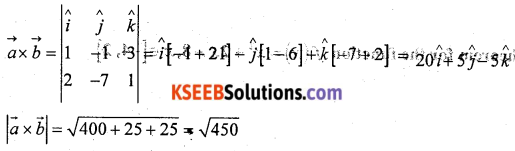Area of the parallelogram = $$|\vec{a} \times \vec{b}|$$ = √450 sq units.

Question 23.
Find the vector and Cartesian equation of the line that passes through the points (3, -2, 5) and (3, -2, 6).
Solution:
Let a and b be the position vectors of points (3, -2, -5), and (3, -2, 6) respectively.
$$\vec{a}=3 \hat{i}-2 \hat{j}-5 \hat{k}$$ and $$b=3 \hat{i}-2 \hat{j}+6 \hat{k}$$
We know that the vector equation of a line passing through the points having position vectors a and b is $$r=\vec{a}+\lambda(\vec{b}-\vec{a})$$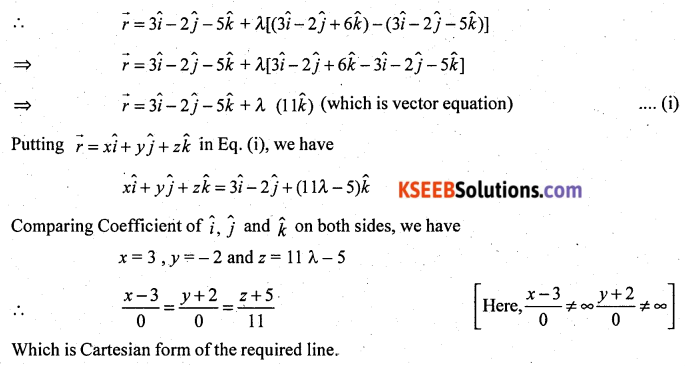Question 24.
Find the probability distribution of the number of heads in two tosses of a coin.
Solution:
When one coin is tossed twice, the sample space is
S = {HH, HT, TH, TT}.
Let X denotes, the number of heads in any outcome in S,
X(HH) = 2, X(HT) = 1, X(TH) = 1 and X(TT) = 0
Therefore, X can take the value of 0, 1 or 2. It is known that
P(HH) = P(HT) = P(TH) = P(TT) = $$\frac{1}{4}$$
P(X = 0) = P (tail occurs on both tosses) = P({TT}) = $$\frac{1}{4}$$
P(X = 1) = P(one head and one tail occurs) = P({TH, HT}) = $$\frac{2}{4}$$ = $$\frac{1}{2}$$
and P(X = 2) = P (head occurs on both tosses) = P({HH}) = $$\frac{1}{4}$$
Thus, the required probability distribution is as follows.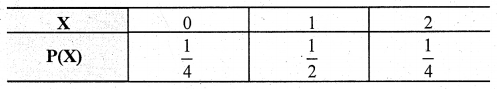Part – C

Anstver any TEN questions: (10 × 3 = 30)

Question 25.
Show that the relation R in R (set of real numbers) is defined as R = {(a, b) : a ≤ b} is reflexive and transitive but not symmetric.
Solution:
a ≤ a is always true
∴ (a, a) R a R
∴ R is reflexive
Let (a, b) ∈ R ⇒ a ≤ b which does not imply b ≤ a
∴ (b, a) ∉ R
∴ R is not symmetric
Let (a, b) ∈ R and (b, c) ∈ R
⇒ a ≤ b and b ≤ c
⇒ a ≤ c
⇒ (a, c) ∈ R
∴ R is transitiveQuestion 26.
Write $$\tan ^{-1}\left(\frac{\sqrt{1+x^{2}}-1}{x}\right)$$, x ≠ 0 in the simplest form.
Solution: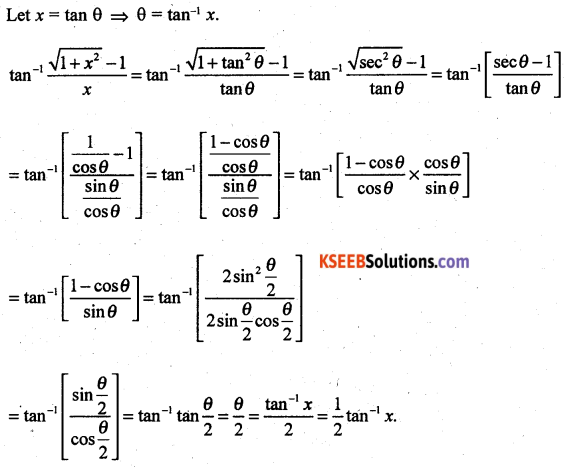Question 27.
If A and B are symmetric matrices of the same order, then show that AB is symmetric if and only if AB = BA.
Solution:
Let A and B are symmetric
∴ A’ = A and B’ = B
Let AB is symmetric
∴ (AB)’ = AB, B’A’ = AB, BA = AB
Conversely, Let AB = BA
(AB)’ = B’A’ = BA = AB
∴ AB is symmetric

Question 28.
Differentiate (log x) cos x with respect to x.
Solution:
Let cos x
y = (log x) cos x
log y = log (log x)
log y = cos x (log x)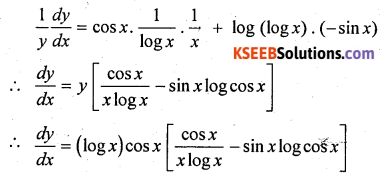Question 29.
Differentiate sin2 x with respect to ecos x.
Solution: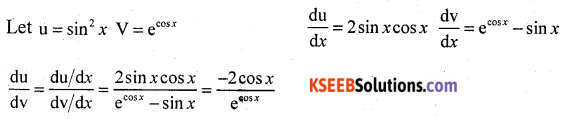Question 30.
Find two positive numbers x and y such that x + y = 60 and xy3 is maximum.
Solution:
Let the two numbers be x, y and P = xy3
Given x + y = 60 ⇒ x = 60 – y
On putting this value in P = xy3, we get
P = (60 – y) y3 ⇒ P = 60y3 – y4
On differentiating twice w.r.t. y, we get
$$\frac{d P}{d y}$$ = 180y2 – 4y3
and $$\frac{d^{2} P}{d y^{2}}$$ = 360y – 12y2
For maxima, we must have $$\frac{d P}{d y}$$ = 0
⇒ 180y2 – 4y3 = 0
⇒ 4y2(45 – y) = 0
⇒ y = 0, 45
But y ≠ 0, so y = 45
At y = 45, $$\left(\frac{d^{2} P}{d y^{2}}\right)_{y=45}$$ = 360 × 45 – 12 × (45)2
=16200 – 24300
= -8100 < 0
⇒ P has afocal maxima at y = 45
∴ By second derivative test, x = 45 is a point of local maxima of P. Thus, the function xy3 is maximum when y = 45 and x = 60 – 45 = 15.
Hence, the required numbers are 15 and 45.Question 31.
Evaluate $$\int \frac{2 x}{x^{2}+3 x+2} d x$$
Solution: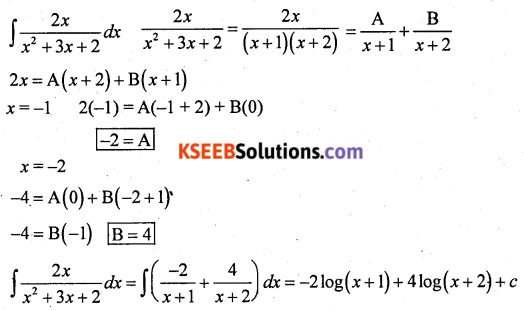Question 32.
Evaluate ∫ex sin x dx.
Solution: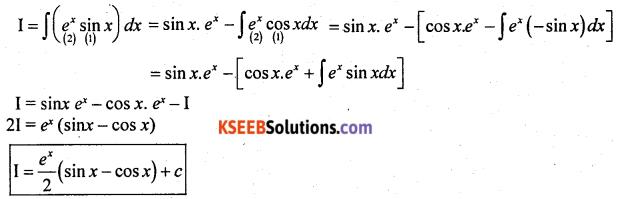Question 33.
Find the area of the region bounded by the curve y2 = 4x, y-axis and the line y = 3.
Solution: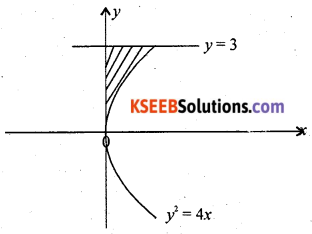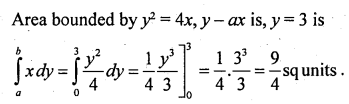Question 34.
Form the differential equation of the family of circles having a centre on y-axis and radius 3 units.
Solution:
The equation of the family circles having centre on y-axis and radius 3 unit is
x2 + (y – b)2 = 9 …….(i)
On differentiating Eq. (i) w.r.t x, we get
2x + 2(y – b) y’ = 0
⇒ y – b = $$-\frac{x}{y^{\prime}}$$ ……. (ii)
On substituting this value of (y – b) in Eq. (i) we get
$$x^{2}+\left(-\frac{x}{y^{\prime}}\right)^{2}=9$$
⇒ x2[(y’)2 + 1] = 9(y’)2
⇒ (x2 – 9)(y’)2 + x2 = 0
which is the required differential equation.Question 35.
Find x, such that the four points A (3, 2, 1), B(4, x, 5), C(4, 2, -2) and D (6, 5, -1) are coplanar.
Solution: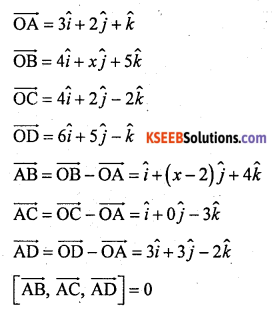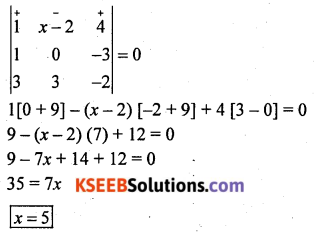Question 36.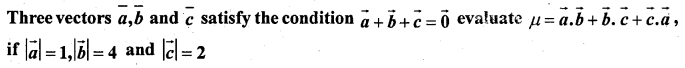Solution: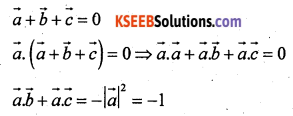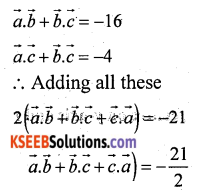Question 37.
Find the shortest distance between the lines $$\vec{r}=\hat{i}+2 \hat{j}+\hat{k}+\lambda(\hat{i}-\hat{j}+\hat{k})$$ and $$\vec{r}=2 \hat{i}-\hat{j}-\hat{k}+\mu(2 \hat{i}+\hat{j}+2 \hat{k})$$
Solution: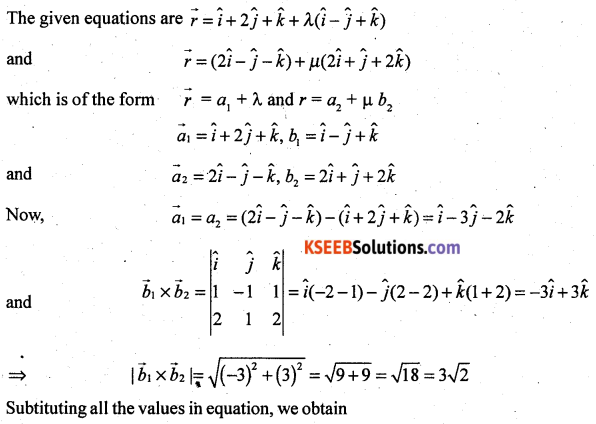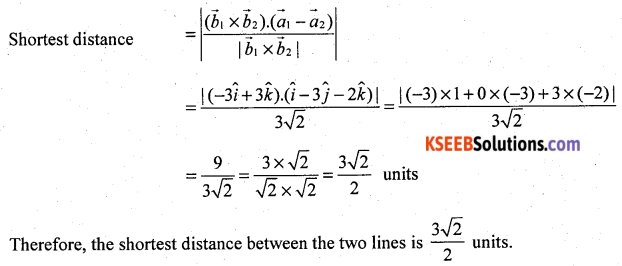Question 38.
Given that the two numbers appearing on throwing two dice are different. Find the probability of the event ‘the sum of numbers on the dice is 4’.
Solution:
When dice is thrown, number of observations in the sample space S = 6 × 6 = 36 (equally likely sample events)
i.e., n(S) = 36
Let E : set of numbers in which numbers appearing on the two dice are different
Then, E = {(1, 3), (2, 2), (3, 1)}
⇒ n(E) = 3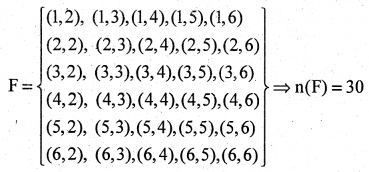n(F) = 30
Here, F contains all points of S except {(1, 1), (2, 2), (3, 3),(4, 4), (5, 5), (6, 6)}
E ∩ F = {(1, 3), (3, 1)}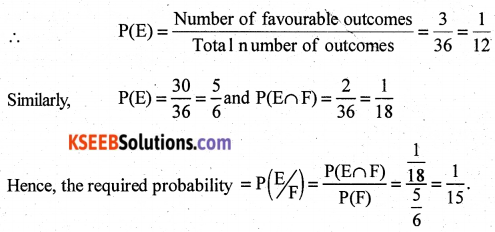Part – D

Answer any SIX questions: (6 × 5 = 30)

Question 39.
Let f : N → R be a function defined as f(x) = 4x2 + 12x + 15. Show that f : N → S, where S is the range of f, is invertible. Find the inverse of f.
Solution: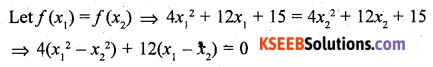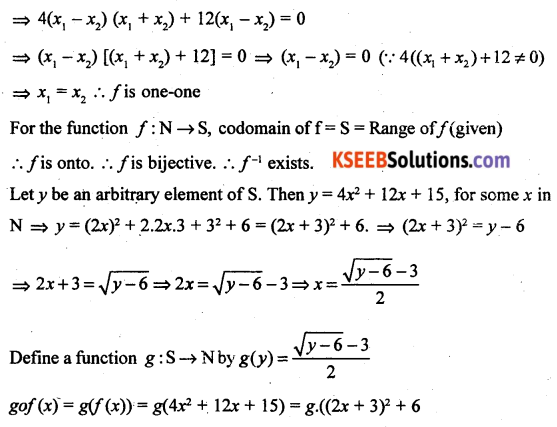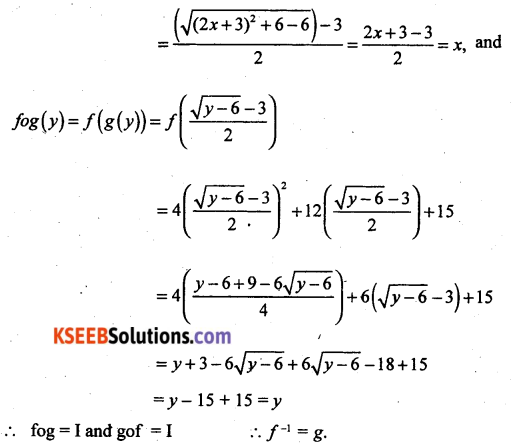Question 40.
If A = $$\left[\begin{array}{lll}1 & 0 & 2 \\0 & 2 & 1 \\2 & 0 & 3\end{array}\right]$$ prove that A3 – 6A2 + 7A + 2I = 0.
Solution: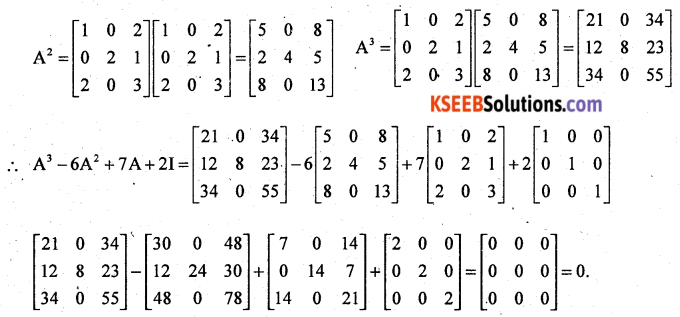Question 41.
Solve the following system of linear equations by matrix method.
x – y + 2z = 1, 2y – 3z = 1 and 3x – 2y + 4z = 2.
Solution: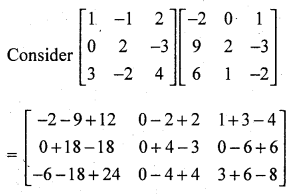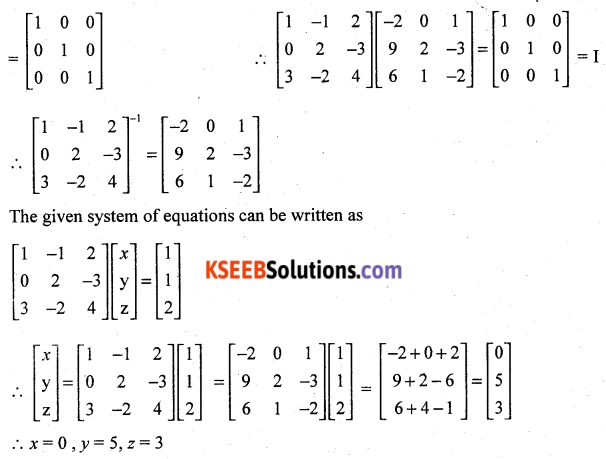Question 42.
If y = (tan-1 x)2. Show that (x2 + 1)2 y2 + 2x(x2 + 1) y1 = 2.
Solution: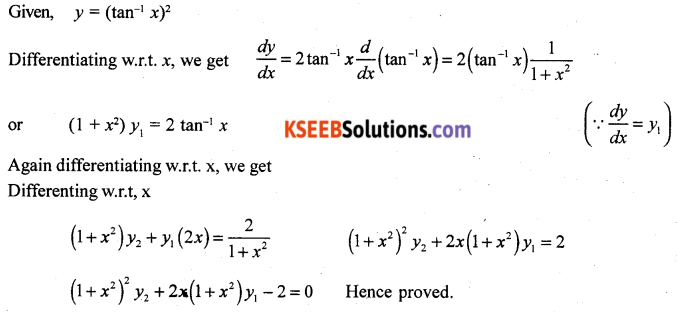Question 43.
The length x of a rectangle is decreasing at the rate of 5 cm/minute and the width y is increasing at the rate of 4 cm/minute when x = 8 cm and y = 6 cm, find the rate of change of
(i) The perimeter and
(ii) The are of the rectangle.
Solution:
At any instant of time t, let length, breadth, perimeter and area of the rectangle are x, y, P and A respectively, then
P = 2(x + y) and A = xy …… (i)
It is given that $$\frac{d x}{d t}$$ = -5 cm/mm and $$\frac{d y}{d t}$$ = -5 = 4 cm/min
(-ve sign shows that the length is decreasing)
(i) Now, P = 2(x + y).
On differentiating w.r.t. t, we get Rate of change of pert meter
$$\frac{d P}{d t}=2\left(\frac{d x}{d t}+\frac{d y}{d t}\right)$$
= 2(-5 + 4) cm/min
= -2 cm/min [∴ $$\frac{d x}{d t}$$ = -5 and $$\frac{d y}{d t}$$ = 4]
Hence, perimeter of the rectangle is decreasing (-ve sign) at the rate of 2 cm/min.
(ii) Here, area of rectangle A = xy.
On differentiating w.r.t. t, we get
Rate of change $$\frac{d A}{d t}=x \frac{d y}{d t}+y \frac{d x}{d t}$$
= 8 × 4 + 6 × (5) [∴ $$\frac{d x}{d t}$$ = -5 and $$\frac{d y}{d t}$$ = 4]
= 32 – 30
= 2 cm2/min
Hence, area of the rectangle is increasing at the rate of 2 cm2/min.
Note: It rate of change is increasing, we take positive sign and if the rate of change is decreasing, then we take a negative sign.Question 44.
Find the integral of $$\sqrt{x^{2}-a^{2}}$$ with respect to x and hence evaluate $$\int \sqrt{x^{2}-8 x+7}$$ dx
Solution: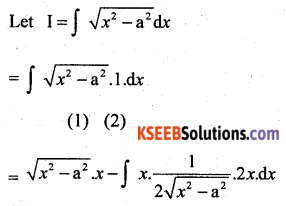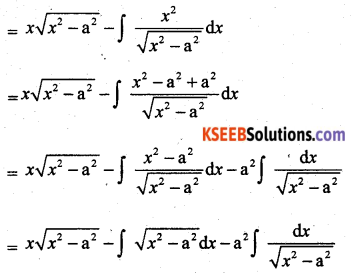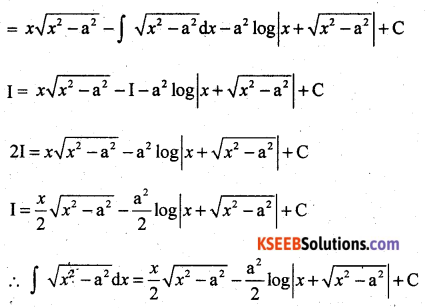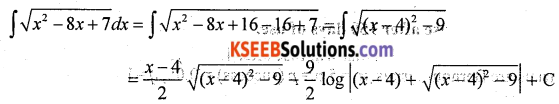Question 45.
Using integration find the area of the triangular region whose sides have the equations y = 2x + 1, y = 3x + 1 and x = 4.
Solution:
Given equation of sides of the triangle are y = 2x + 1, y = 3x + 1 and x = 4.
On solving these equations, we obtain the vertices of the triangle as A(0, 1), B(4, 13) and C(4, 9).
Required area (shown in the shaded region)
= Area (OLBAO) – Area (OLCAO)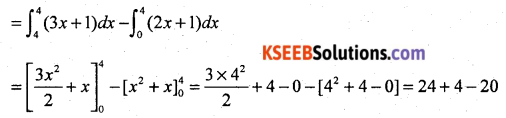= 8 sq.units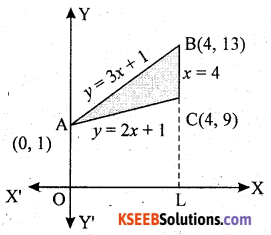Question 46.
Solve the differential equation $$\cos ^{2} x \frac{d y}{d x}+y=\tan x\left(0 \leq x<\frac{\pi}{2}\right)$$
Solution: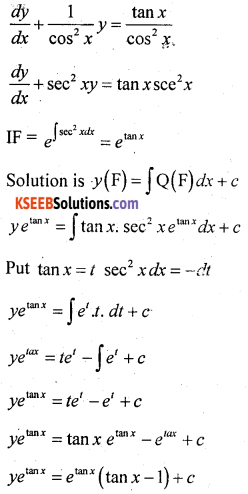Question 47.
Derive the equation of a plane perpendicular to a given vector and passing through a given point both in vector and Cartesian form.
Solution:
Vector Form
Let a plane pass through a point A with position vector $$\overrightarrow{\mathrm{a}}$$ and perpendicular to the vector $$\overrightarrow{\mathrm{N}}$$
Let $$\overrightarrow{\mathrm{r}}$$ be the position vector of any point P(x, y, z) in the plane.
Then the point P lies in the plane if and only if $$\overline{\mathrm{AP}}$$ is perpendicular to $$\overrightarrow{\mathrm{N}}$$
i.e. $$\overline{\mathrm{AP}} \cdot \overline{\mathrm{N}}=0$$
But $$\overline{\mathrm{AP}}=\overrightarrow{\mathrm{r}}-\overrightarrow{\mathrm{a}}$$
Therefore $$(\overrightarrow{\mathbf{r}}-\overrightarrow{\mathrm{a}}) \cdot \overrightarrow{\mathrm{N}}=0$$ …….. (1)
This is the vector equation of the plane.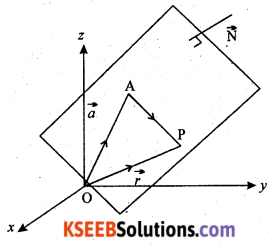Cartesian form
Let the given point A be (x1, y1, z1), P be (x, y, z) and direction ratios of
$$\overline{\mathrm{N}}$$ are A, B and C. Then
$$\overrightarrow{\mathrm{a}}=x_{1} \hat{\mathrm{i}}+\mathrm{y}_{1} \hat{\mathrm{j}}+\mathrm{z}_{1} \hat{\mathrm{k}}, \quad \overrightarrow{\mathrm{r}}=x \hat{\mathrm{i}}+\mathrm{y} \hat{\mathrm{j}}+\mathrm{z} \hat{\mathrm{k}}$$ and $$\overline{\mathrm{N}}=\mathrm{A} \hat{\mathrm{i}}+\mathrm{B} \hat{\mathrm{j}}+\mathrm{C} \hat{\mathrm{k}}$$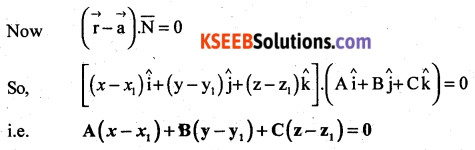Question 48.
The probability that a bulb produced by a factory will fuse after 150 days of use is 0.05. Find the probability that out of 5 such bulbs.
(i) none
(ii) not more than one
(iii) more than one will fuse after 150 days of use.
Solution:
Let X represents the number of bulbs that will fuse after 150 days of use in an experiment of 5 trials. The trials are Bernoulli trials, n = 5p = p (success) = 0.05, q = 1 – p = 1 – 0.05 = 0.95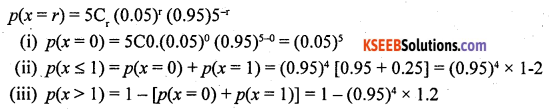Part – E

Answer ary ONE question: (1 × 10 = 10)

Question 49(a).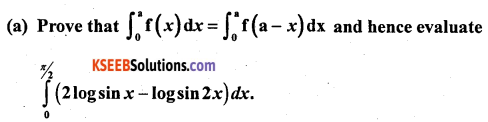Solution: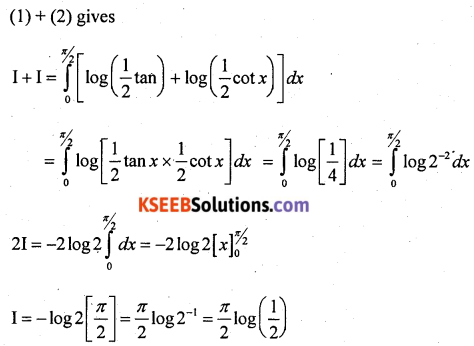Question 49(b).
Show that $$\left|\begin{array}{ccc}x & x^{2} & y z \\y & y^{2} & z x \\z & z^{2} & x y\end{array}\right|$$ = (x – y) (y – z) (z – x) (xy + yz + zx)
Solution: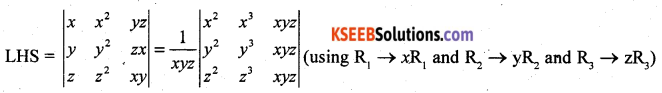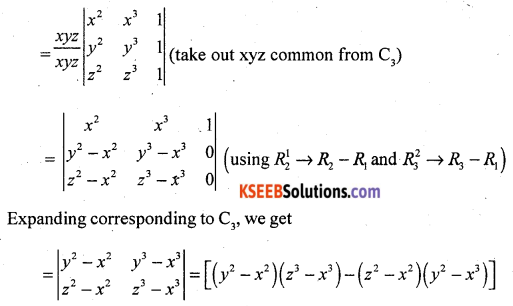= (y + x)(y – x)(z – x)(z3 + x2 + xz) – (z + x)(z – x)(y – x)(y2 + x2 + xy)
= (y – x)(z – x)[(y – x)(z3 + x2 + xz) – (z + x)(y2 + x2 + xy)]
= (y – x)(z – x) [(yz2 + yx2 + xyz + xz2 + x3 + x2z – zy2 – zx2 – xyz – xy2 – x3 – x2y]
= (y – x)(z – x)[yz2 + zy2 + xz2 – xy2]
= (y – x) (z – x) [yz (z – y) + x (z – y)(z + y)]
= (y – x) (z – x) [(z – y) (xy + yz + zx)]
= (x – y) (y – z) (z – x) (xy + yz + zx)
= RHS
Hence proved.

Question 50(a).
Minimize and maximize z = 600x + 400y
Subject to the constraints:
x + 2y ≤ 12
2x + y ≤ 12
4x + 5y ≥ 20 and x ≥ 0, y ≥ 0 by graphical method.
Solution:
x + 2y = 12
y = 0 ⇒ x = 12 ∴ P (12, 0)
x = 0 ⇒ y = 6 ∴ D (0, 6)
2x + 2y = 12
y = 0 ⇒ x = 6 ∴ B (6, 0)
x = 0 ⇒ y = 12 ∴ F (0, 12)
4x + 5y = 20
y = 0 ⇒ x = 5 ∴ A (5, 0)
x = 0 ⇒ y = 4 ∴ E (0, 4)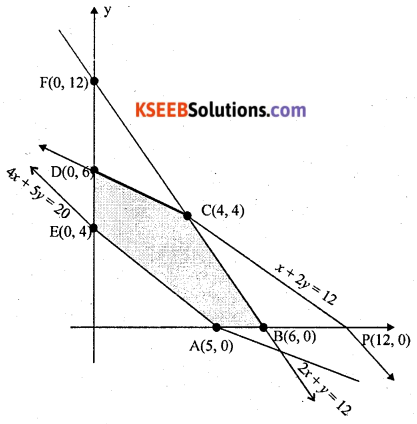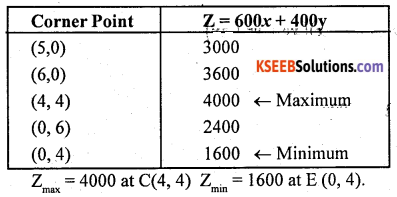Question 50(b).
Find the value of k, if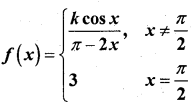Solution: